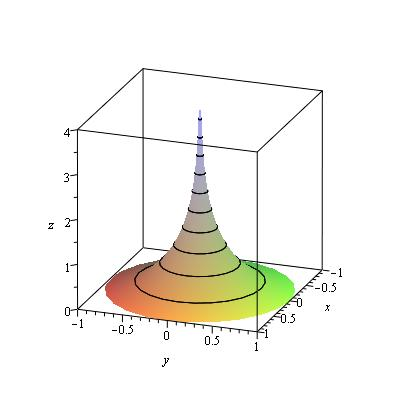# Volume of a Parametrised Surface

## Homework Statement

Let C be the parametrised surface given by

Φ(t,θ)=(cosθ/cosht, sinθ/cosht,t−tanht), for 0≤t and 0≤θ<2π

Let V be the region in R3 between the plane z = 0 and the surface C.

Compute the volume of the region V .

## The Attempt at a Solution

I thought I needed to perform a change of variables; changing from x,y,z to theta,t,z.

I tried to find the Jacobean for this, but it came to zero. I'm pretty sure it was wrong in the first place, but I have no idea what to do otherwise. Your assistance would be greatly appreciated.

Dick
Homework Helper
It's a surface of revolution. To visualize what it looks like, figure out what cross sections of constant ##t## are.

LCKurtz
Homework Helper
Gold Member
Here's a picture if that helps you. In the picture ##0\le t \le 5##.#### Attachments

Thanks heaps! That’s very helpfulI’m a little uncertain about how to setup the integral to calculate the volume though. Am I correct in needing to perform a change of variables? If so, what am I doing incorrectly that produces a Jacobean of zero?

ThanksDick
Thanks heaps! That’s very helpfulThanks